## tkinter -- Canvas(4)

绘制弧形

代码；

```# 绘制弧形
import tkinter as tk
root = tk.Tk()
# 创建一个 Canvas，设置其背景色为白色
cv = tk.Canvas(root, bg='white')
cv.create_arc((10,10,110,110),)
cv.pack()
root.mainloop()
# 使用默认参数创建一个 ARC，结果为90度的扇形```

结果:设置弧形的样式

创建 create_arc

代码:

```import tkinter as tk
root = tk.Tk()
# 创建一个 Canvas，设置其背景色为白色
cv = tk.Canvas(root, bg='white')
d = {1:tk.PIESLICE, 2:tk.CHORD, 3:tk.ARC}
for i in d:
cv.create_arc((10, 10 + 60*i, 110, 110+60*i),style=d[i])
print(i, d[i])
cv.pack()
root.mainloop()
# 使用三种样式，分别创建了扇形、弓形和弧形```

结果: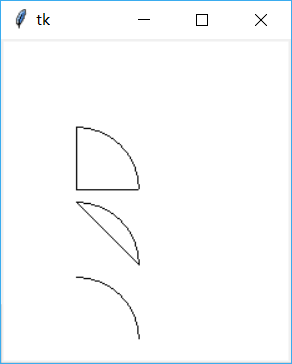设置弧形的角度

使用 start/extent 指定起始角度与偏移角度

代码:

```import tkinter as tk
root = tk.Tk()
# 创建一个 Canvas，设置其背景色为白色
cv = tk.Canvas(root, bg='white')
d = {1:tk.PIESLICE, 2:tk.CHORD, 3:tk.ARC}
for i in d:
cv.create_arc((10, 10 + 60*i, 110, 110+60*i),
style=d[i],  # 指定样式
start=30,  # 指定起始角度
extent=30,  # 指定角度偏移量
)
print(i, d[i])
cv.pack()
root.mainloop()
# 使用三种样式，start 指定起始角度；extent 指定角度偏移```

结果:绘制位图

使用 bitmap 创建位图 create_bitmap

代码:

```import tkinter as tk
root = tk.Tk()
# 创建一个 Canvas，设置其背景色为白色
cv = tk.Canvas(root, bg='white')
d = {1:'error', 2:'info', 3:'question', 4:'hourglass'}
for i in d:
cv.create_bitmap((20*i,20*i),bitmap=d[i])
cv.pack()
root.mainloop()
# 使用 bitmap 属性来指定位图的名称，这个函数的第一个参数为一个点(x,y)指定位图存放位置的左上位置```

结果:绘制 GIF 图像

创建 gif 图像 create_image

代码:

```import tkinter as tk

root = tk.Tk()
# 创建一个 Canvas，设置其背景色为白色
cv = tk.Canvas(root, bg='white')
img = tk.PhotoImage(file='444.gif')
cv.create_image((150,150), image=img)
cv.pack()
root.mainloop()```

结果:先使用 PhotoImage 创建 GIF 图像，再将 image 属性来设置为新创建的 img

绘制直线

创建带箭头的直线 create_line

代码:

```import tkinter as tk

root = tk.Tk()
# 创建一个 Canvas，设置其背景色为白色
cv = tk.Canvas(root, bg='white')
d = [(0,'none'),(1, 'first'),(2,'last'),(3,'both')]
for i in d:
cv.create_line(
(10, 10 + i*20, 110, 110 + i*20),  # 设置直线的起始、终点
arrow=i,  # 设置直线是否使用箭头
arrowshape='40 40 10',  # 设置箭头的形状(填充长度，箭头长度，箭头宽度

)
cv.pack()
root.mainloop()```

结果:使用 arrow 属性来控制是否显示箭头

直线的 joinstyle 属性

创建直线，使用 joinstyle 属性

代码:

```import tkinter as tk
root = tk.Tk()
# 创建一个 Canvas，设置其背景色为白色
cv = tk.Canvas(root, bg='white')
d = [(0,'none','bevel'),(1, 'first','miter'),(2,'last','round'),(3,'both','round')]
for i in d:
cv.create_line(
(10, 10 + i*20, 110, 110 + i*20),  # 设置直线的起始、终点
arrow=i,  # 设置直线是否使用箭头
arrowshape='8 10 3',  # 设置箭头的形状(填充长度，箭头长度，箭头宽度
joinstyle=i,
)
cv.pack()
root.mainloop()```

结果: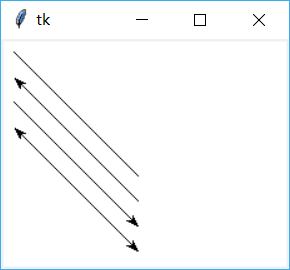将直线的属性 joinstyle 分别设置为 bevel/miter/round，测试其效果

绘制椭圆

绘制椭圆，使用 create_oval 属性

代码:

```import tkinter as tk
root = tk.Tk()
# 创建一个 Canvas，设置其背景色为白色
cv = tk.Canvas(root, bg='white')
# 创建一个长200，宽100的椭圆
cv.create_oval((10,10,210,110), fill='red')
cv.pack()
root.mainloop()```

结果:指定椭圆的长和宽，圆是长和宽相等的特殊情况

创建多边形

创建多边形（三角形）

代码:

```import tkinter as tk
root = tk.Tk()
# 创建一个 Canvas，设置其背景色为白色
cv = tk.Canvas(root, bg='white')
# 创建一个直角三角形
cv.create_polygon((10,10,10,200,100,200), fill='red')
cv.pack()
root.mainloop()```

结果: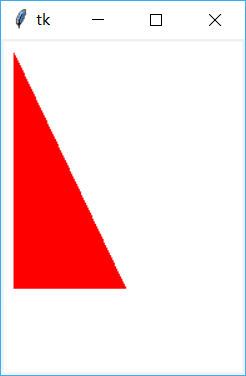指定三个点的坐标，三个点坐标必须满足三角形的定义

修饰图形

创建多边形 create_ploygon（三角形）

代码:

```import tkinter as tk
root = tk.Tk()
# 创建一个 Canvas，设置其背景色为白色
cv = tk.Canvas(root, bg='white')
# 创建一个直角三角形
cv.create_polygon((10,10,10,200,100,200),
smooth=True,
splinesteps=0,
fill='red')
cv.pack()
root.mainloop()```

结果: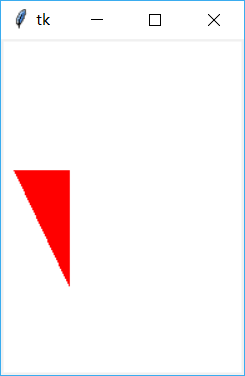smooth/splinesteps 用来修改绘制的图形

绘制文字

使用文字 create_text

代码:

```import tkinter as tk
root = tk.Tk()
# 创建一个 Canvas，设置其背景色为白色
cv = tk.Canvas(root, bg='white')
# 创建一个文字对象，默认设置为居中对齐
cv.create_text((10,10),text='hello text',anchor='w')
cv.pack()
root.mainloop()```

结果: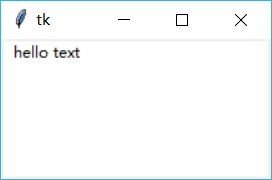使用 anchor 控制文字的位置，使用 justify 控制对齐方式

选中文字

使用文字 create_text

代码:

```import tkinter as tk
root = tk.Tk()
# 创建一个 Canvas，设置其背景色为白色
cv = tk.Canvas(root, bg='white')
# 创建一个文字对象，默认设置为居中对齐
txt = cv.create_text((10,10), text='hello text',anchor='w')
# 设置文本的选中起始位置
cv.select_from(txt, 2)

# 设置文本的选中结束位置
cv.select_to(txt, 5)
cv.pack()
root.mainloop()```

结果:使用 anchor 控制文字的位置，使用 justify 控制对齐方式

创建组件

使用子组件 create_window

代码:

```import tkinter as tk
root = tk.Tk()
# 创建一个 Canvas，设置其背景色为白色
cv = tk.Canvas(root, bg='white')

def printWindow():
print('window')
# 创建一个 Button 对象，默认设置为居中对齐
bt = tk.Button(cv, text='ClickMe', command=printWindow)

#修改 button 在 canvas 上的对齐方式
cv.create_window((10,10), window=bt, anchor='w')

# 新创建的 line 对象与 button 有重叠
cv.create_line(10,10,20,20)

# 新创建的 line 不在 button 之上，即没有重叠
cv.create_line(30,30,100,100)

cv.pack()
root.mainloop()```

结果: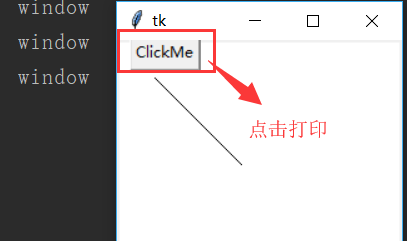使用 anchor 组件在 Canvas 上的位置，默认情况下为居中对齐,这样使用后其它的 item将不能再使用 button 占用的那块区域